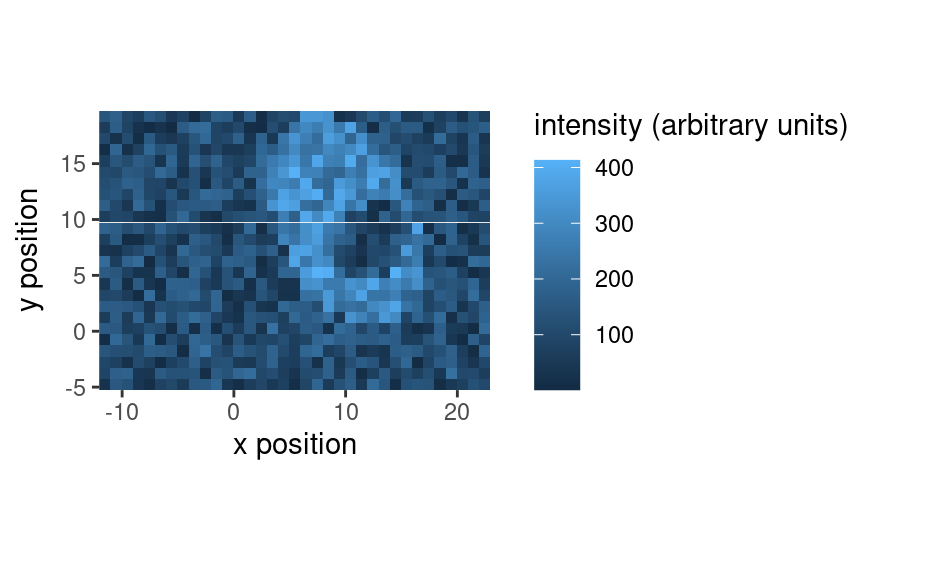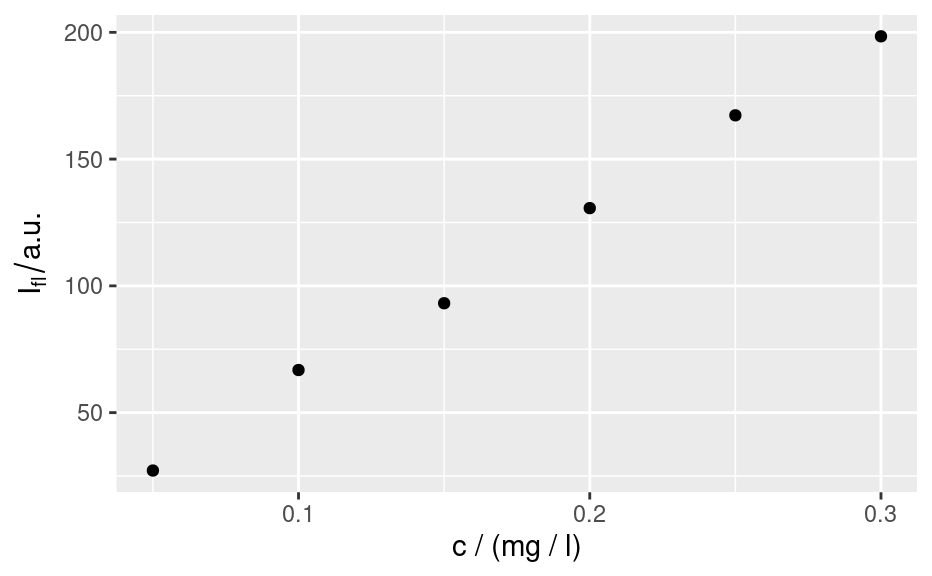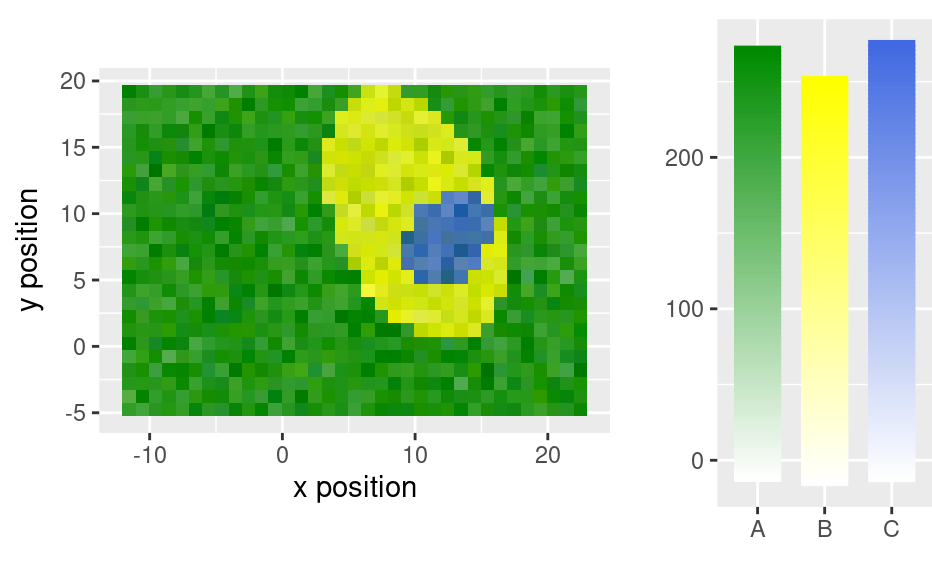These ggplot2-related hyperSpec functions are deprecated and they will be removed in the next release of the package. Now functions from package hySpc.ggplot2 (link) should be used as alternatives to plot hyperSpec objects with ggplot2.

qplotspc(
x,
wl.range = TRUE,
...,
mapping = aes_string(x = ".wavelength", y = "spc", group = ".rownames"),
spc.nmax = hy_get_option("ggplot.spc.nmax"),
map.lineonly = FALSE,
debuglevel = hy_get_option("debuglevel")
)

qplotmap(
object,
mapping = aes_string(x = "x", y = "y", fill = "spc"),
...,
func = mean,
func.args = list(),
map.tileonly = FALSE
)

qplotc(
object,
mapping = aes_string(x = "c", y = "spc"),
...,
func = NULL,
func.args = list(),
map.pointonly = FALSE
)

qplotmixmap(object, ...)

legendright(p, l, legend.width = 8, legend.unit = "lines")

qmixtile(
object,
purecol = stop("pure component colors needed."),
mapping = aes_string(x = "x", y = "y", fill = "spc"),
...,
map.tileonly = FALSE
)

normalize.colrange(x, na.rm = TRUE, legend = FALSE, n = 100, ...)

normalize.range(x, na.rm = TRUE, legend = FALSE, n = 100, ...)

normalize.null(x, na.rm = TRUE, legend = FALSE, n = 100, ...)

normalize.minmax(x, min = 0, max = 1, legend = FALSE, n = 100, ...)

qmixlegend(
x,
purecol,
dx = 0.33,
ny = 100,
labels = names(purecol),
normalize = normalize.colrange,
...
)

colmix.rgb(
x,
purecol,
against = 1,
sub = TRUE,
normalize = normalize.colrange,
...
)

Arguments

x

matrix with component intensities in columns

wl.range

wavelength ranges to plot

...

qmixtile: handed to colmix.rgb()

qmixlegend() and colmix.rgb() hand further arguments to the normalize function

mapping
spc.nmax

maximum number of spectra to plot

map.lineonly

if TRUE, mapping will be handed to ggplot2::geom_line() instead of ggplot2::ggplot().

debuglevel

if > 0, additional debug output is produced

object

matrix to be plotted with mixed colour channels

func

function to summarize the wavelengths, if NULL, only the first wavelength is used

func.args

arguments to func

map.tileonly

if TRUE, mapping will be handed to ggplot2::geom_tile() instead of ggplot2::ggplot().

map.pointonly

if TRUE, mapping will be handed to ggplot2::geom_point() instead of ggplot2::ggplot().

p

plot object

l

legend object

legend.width, legend.unit

size of legend part

purecol

pure component colours, names determine legend labels

na.rm

see base::min()

legend

should a legend be produced instead of normalized values?

n

of colours to produce in legend

min

numeric with value corresponding to "lowest" colour for each column

max

numeric with value corresponding to "hightest" colour for each column

dx

width of label bar

ny

number of colours in legend

labels

component names

normalize

function to normalize the values.

against

value to mix against (for sub = TRUE only, 1 = white, 0 = black)

sub

subtractive color mixing?

Claudia Beleites

Examples

qplotspc(flu)
#> Warning: Function 'qplotspc' is deprecated.
#> Use function 'qplotspc' from package 'hySpc.ggplot2' instead.
#> https://r-hyperspec.github.io/hySpc.ggplot2qplotmap(faux_cell[, , 1200])
#> Warning: Function 'qplotmap' is deprecated.
#> Use function 'qplotmap' from package 'hySpc.ggplot2' instead.
#> https://r-hyperspec.github.io/hySpc.ggplot2qplotc(flu)
#> Warning: Function 'qplotc' is deprecated.
#> Use function 'qplotc' from package 'hySpc.ggplot2' instead.
#> https://r-hyperspec.github.io/hySpc.ggplot2
#> Warning: Intensity at first wavelengh only is used.faux_cell <- faux_cell - spc_fit_poly_below(faux_cell)

qplotmixmap(faux_cell[, , c(800, 1200, 1500)],
purecol = c(A = "green4", B = "yellow", C = "royalblue")
)
#> Warning: Function 'qplotmixmap' is deprecated.
#> Use function 'qplotmixmap' from package 'hySpc.ggplot2' instead.
#> https://r-hyperspec.github.io/hySpc.ggplot2
#> Warning: Removed 300 rows containing missing values (geom_point).